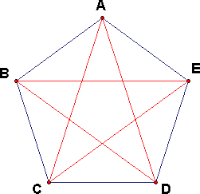# Pentagons and Ratios

Geometry Level 5Each diagonal of the convex pentagon $ABCDE$ cuts off of it a triangle of unit area. Calculate the area of ​​the pentagon $ABCDE$, and express the answer to the three decimal places.

Note: The pentagon need not be regular.

×